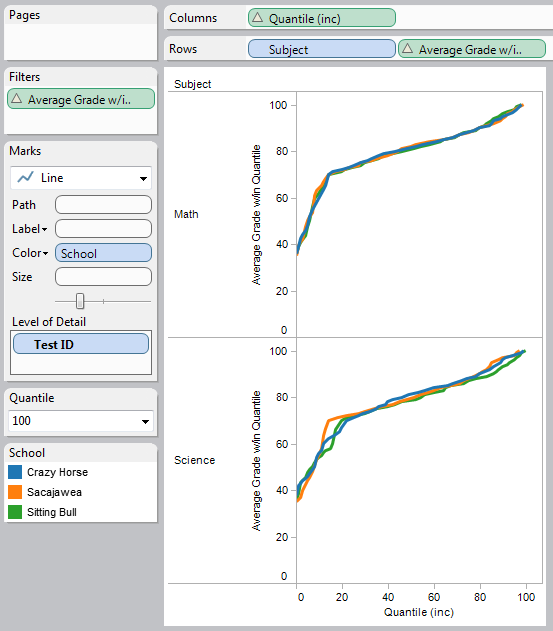# Tag Archives: partitioning# Partitioning by Table Calculations

Here’s a issue on the Tableau forums that shows up at least once per month or so: someone has a data set that they want to compute a measure over, and the measure requires table calculations. Then they want to use the results of those calculations as a discrete value for partitioning other calculations. For example, given a set of student grades, compute each student’s percentile, then show the average of each percentile in the same view. Or a similar idea, only the percentile is based on sum of sales, and you want to show the top 10% and bottom 10% of sales. Continue reading